# Discussion Forum

Discussion Closed This discussion was created more than 6 months ago and has been closed. To start a new discussion with a link back to this one, click here.

## Strange Issue in Nonlinear Viscoelastic Solid Mechanics Simulation

Good Afternoon Everyone,

I am attempting to implement a predictive model for Viscoelastic deformation in COMSOL. To do this, I have derived several models from fundamental continuum mechanics, but I am having trouble with the implementation of my most complicated one---the single-integral, Nonlinear Schapery model.

There are two portions of the Schapery model: elastic and transient. Together, they take the following form: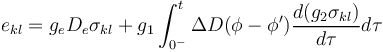In addition, I am modeling the transient portion of the compliance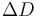using a common power-law model as follows: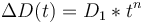The reduced-time terms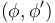are analagous to Time-Temperature-Superposition methods, except by modulating Time with Stress instead. In this case, consider their effect to be scaling the time input for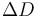according to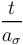.

Attached is the most recent version of my COMSOL model, which is meant to mimic a viscoelastic creep-recovery test that I will be performing in a lab once the COMSOL model is working. The BC's are an axially-fixed top edge, and a boundary load on the bottom edge which corresponds to a step stress input. Fairly simple, and in line with the examples I have found on this forum and in the COMSOL example model files (specifically "bar-necking.mph").

In theory, the elastic portion of the model should be nearly identical to the built-in elastic strain calculated by COMSOL, so I have included an "initial stress/strain" node under a renamed "elastic material" node. This should add or subtract the appropriate difference between the COMSOL elastic strain and what the Schapery model calculates in a Domain ODE Physics node.

The transient convolution integral was somewhat complicated to get working, but at this point it should operate similarly. I have added another Domain ODE node that calculates a running integral, and a second node that multiplies the running integral by the appropriate nonlinear variable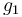. The resulting transient strain is added using another "initial stress/strain" node.

My dilemma is this: the simulation appears to consistently fail at 15 seconds. Every time. No matter how I constrain the geometry or change the solver. I have even tried systematically removing each variable from the transient strain integration Domain ODE equation, and none have changed the resulting simulation death at 15 seconds.

The error is as follows:

• Feature: Time-Dependent Solver 1 (sol1/t1) Nonlinear solver did not converge. Transient Integration Singular matrix.

There are 16200 equations giving NaN/Inf in the matrix rows for the variable comp2.transInt1. at coordinates: (0.00159842,2.11404e-005), (0.00159842,4.22807e-005), (0.00159842,6.34211e-005), (0.00159894,2.11404e-005), (0.00159894,4.22807e-005), ...

There are 16200 equations giving NaN/Inf in the matrix rows for the variable comp2.transInt2. at coordinates: (0.00159842,2.11404e-005), (0.00159842,4.22807e-005), (0.00159842,6.34211e-005), (0.00159894,2.11404e-005), (0.00159894,4.22807e-005), ...

There are 16200 equations giving NaN/Inf in the matrix rows for the variable comp2.transInt3. at coordinates: (0.00159842,2.11404e-005), (0.00159842,4.22807e-005), (0.00159842,6.34211e-005), (0.00159894,2.11404e-005), (0.00159894,4.22807e-005), ...

There are 16200 equations giving NaN/Inf in the matrix rows for the variable comp2.transInt4. at coordinates: (0.00159842,2.11404e-005), (0.00159842,4.22807e-005), (0.00159842,6.34211e-005), (0.00159894,2.11404e-005), (0.00159894,4.22807e-005), ...

There are 16200 equations giving NaN/Inf in the matrix rows for the variable comp2.transInt5. at coordinates: (0.00159842,2.11404e-005), (0.00159842,4.22807e-005), (0.00159842,6.34211e-005), (0.00159894,2.11404e-005), (0.00159894,4.22807e-005), ...

There are 16200 equations giving NaN/Inf in the matrix rows for the variable comp2.transInt6. at coordinates: (0.00159842,2.11404e-005), (0.00159842,4.22807e-005), (0.00159842,6.34211e-005), (0.00159894,2.11404e-005), (0.00159894,4.22807e-005), ...

and similarly for the degrees of freedom, NaN/Inf in the matrix columns.

Time : 15

In Transient Integration:

Last time step is not converged.

Clearly something is causing an undefined value, but I am not sure where in the equation this is occurring. Each of the variables is continuously defined for the stresses I am seeing in my 2D plot, and the time is clearly not undefined. I have attempted a nearly incomprehensible number of permutations to try and get past 15 seconds simulation time. Is there any reason that the simulation would suddenly become undefined/NaN at 15 seconds, no matter what the applied load is? In particular, is there a feature of using the time variable 't' as I have in the equation that could be causing the instability? The nonlinear parameters are directly from a paper that I am attempting to reproduce strain values for.

Any help would be very welcome, and sincerely appreciated. I've been stuck here for the last few weeks, and have run myself ragged looking for a solution. Thank you in advance!

1 Reply Last Post 15-Jan-2019, 10:53 AM GMT-5

Posted: 10 months ago

For those who encounter this error:

I reset my solver to default, and the system moved past 15s. Befuddled, I went and stepped through each of the settings, slowly returning each setting to the ones I had previously chosen.

The issue appeared to occur when I had changed the "Amplification for High Frequency" to a lower value (default is 0.75, I had set it to 0.1 due to the extreme nonlinearity of my material model). The simulation still failed, and I was able to find that at the initial time (t=0) my integral was causing an undefined stiffness matrix.

Changing that setting, and additionally adding the term "eps" to my time integral varible "t" solved both issues. The "eps" is necessary because at the initial time (zero, in my case) my power law is raising zero to some power, which COMSOL does not allow.

Now, for some reason the strain is not properly showing creep properties (i.e. an initial elastic strain response, and a slower transient response), though that could be an issue with the parameterization of my model. I will investigate further and check back in if there are more errors (though if the issue is different enough, I will make a new thread).

Note that while COMSOL employees may participate in the discussion forum, COMSOL® software users who are on-subscription should submit their questions via the Support Center for a more comprehensive response from the Technical Support team.